# One mole of an ideal gas, initially at 303.15 K (30°C) and 1 bar, is changed to 403.15 K (130°C) and 10 bar by three different mechanically reversible processes:The gas is first heated at constant volume until its temperature is 403.15 K ( 1 30°C); then it is compressed isothermally until its pressure is 10 bar.The gas is first heated at constant pressure until its temperature is 403.15 K (130°C); then it is compressed isothermally to 10 bar.The gas is first compressed isothermally to 10 bar; then it is heated at constant pressure to 403.15 K (130°C).Calculate Q, W, U, and AH

Question
132 views

One mole of an ideal gas, initially at 303.15 (30°C) and bar, is changed to 403.15 (130°C) and 10 bar by three different mechanically reversible processes:

The gas is first heated at constant volume until its temperature is 403.15 ( 1 30°C); then it is compressed isothermally until its pressure is 10 bar.

The gas is first heated at constant pressure until its temperature is 403.15 (130°C); then it is compressed isothermally to 10 bar.

The gas is first compressed isothermally to 10 bar; then it is heated at constant pressure to 403.15 (130°C).

Calculate Q, W, U, and AH

check_circle

Step 1

Given data:

Number of moles of an ideal gas = 1

Initial temperature (T1) = 303.15 K

Final temperature (T) = 403.15 K

Initial pressure (P1) = 1 bar

Final pressure (P2) = 10 bar

The ideal gas is changed from state 1 to state 2 by three different mechanically reversible processes.

Step 2
1. In the first case, gas is first heated at constant volume until its temperature is 403.15 K and then compressed isothermally until its pressure is 10 bar.

For an ideal gas, the values of specific heat at constant volume (Cv) and at constant pressure (Cp) are 2.5 R and 3.5 R respectively.

Where R is the universal gas constant.

For a constant volume process, heat is calculated as follows: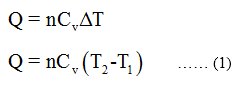Where n represents the number of moles

Cv represents the specific heat at constant volume

T1 represents the initial temperature

T2 represents the final temperature

The value of universal gas constant (R) = 8.314 J/Mol × K

Plugging the values in equation 1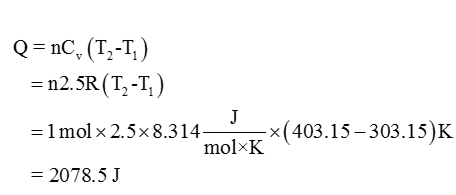Step 3

Further gas is compressed isothermally (temperature constant) until pressure is 10 bar.

Work done (W) for this process is calculates as follows: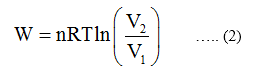The ratio of volume can be written in terms of pressure as follows:

Since the gas is ideal and the process is isothermal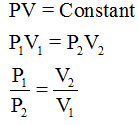Therefore, equation 2 becomes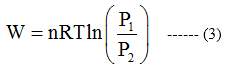Plugin the values in equation 3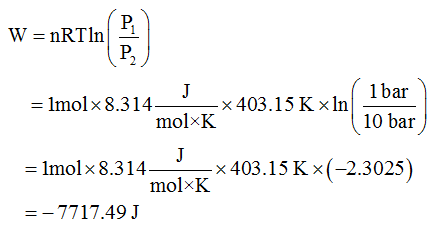...

### Want to see the full answer?

See Solution

#### Want to see this answer and more?

Solutions are written by subject experts who are available 24/7. Questions are typically answered within 1 hour.*

See Solution
*Response times may vary by subject and question.
Tagged in